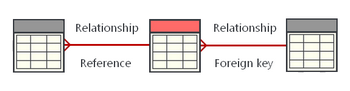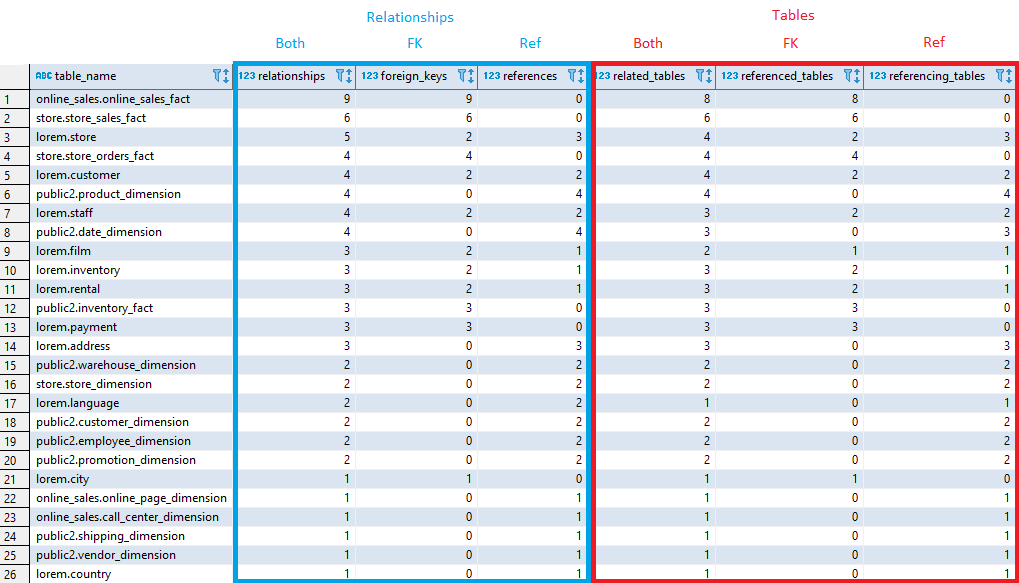## List tables with most relationships in Vertica database

Article for:

Query below lists tables with most relationships (both foreign keys and FK references from other tables).## Query

``````with rel as (
select distinct table_schema || '.' || table_name as table_name,
reference_table_schema || '.' || reference_table_name
as referenced_table,
null as referencing_table,
constraint_id
from v_catalog.foreign_keys
union all
select distinct reference_table_schema || '.' || reference_table_name,
null,
table_schema || '.' || table_name as table_name,
constraint_id
from v_catalog.foreign_keys
)
select table_name,
count(*) as relationships,
count(referenced_table) as foreign_keys,
count(referencing_table) as references,
count(distinct coalesce(referenced_table, referencing_table))
as related_tables,
count(distinct referenced_table) as referenced_tables,
count(distinct referencing_table) as referencing_tables
from rel
group by table_name
order by relationships desc;
``````

## Columns

• table_name - name of table with schema name
• relationships - number of relationships (FKs and FK references)
• foreign_keys - number of foreign keys in a table
• references - number of FK references from other tables
• related_tables - number of related tables
• referenced_tables - number of different tables referenced with FKs (multiple FK's can refers to one table, so number of foreign keys might be diffrent than number of referenced tables)
• referencing_tables - number of diffrent tables that are refering to this table (similar note like as above)

## Rows

• One row represents one table in a database
• Scope of rows: all tables in database with any relationship
• Ordered by number of relationships (foreing keys and references) from the ones with the most

## Sample results